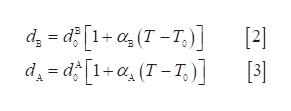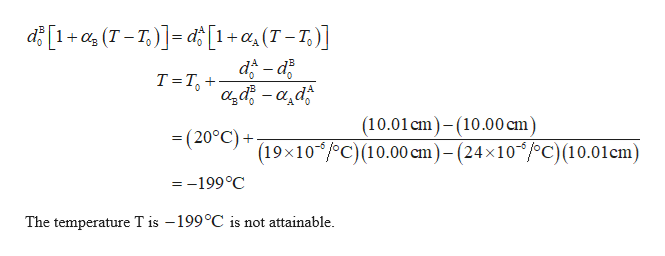# A brass ring of diameter 10.00 cm at 20.0°C is heated and slipped over an aluminum rod of diameter 10.01 cm at 20.0°C. Assuming the average coefficients of linear expansion are constant, (a) to what temperature must the combination be cooled to separate the two metals? Is that temperature attainable? (b) What if the aluminum rod were 10.02 cm in diameter?

Question
43 views

A brass ring of diameter 10.00 cm at 20.0°C is heated and slipped over an aluminum rod of diameter 10.01 cm at 20.0°C. Assuming the average coefficients of linear expansion are constant, (a) to what temperature must the combination be cooled to separate the two metals? Is that temperature attainable? (b) What if the aluminum rod were 10.02 cm in diameter?

check_circle

Step 1

(a) For the ring to be removed from the rod, the diameters of the brass and the aluminium is same at the final temperature.

Step 2

The expression for the diameter of the brass and the aluminium ishelp_outlineImage Transcriptionclosed, = d? [1+ a, (T - T,)]  d̟ = d [1+a, (T – T,)] 3)  fullscreen
Step 3

Use equation (2) and (3) in (1) to sol...help_outlineImage Transcriptionclosed [1+cz (T = T,)]= d[1+a,(T - T,)] da – d? a,d, - a,d T=T, +• 0, (10.01cm)-(10.00 cm) (19×10*/°C)(10.00 cm)– (24×10´/°C)(10.01cm) = (20°C) + = -199°C The temperature T is –199°C is not attainable. fullscreen

### Want to see the full answer?

See Solution

#### Want to see this answer and more?

Solutions are written by subject experts who are available 24/7. Questions are typically answered within 1 hour.*

See Solution
*Response times may vary by subject and question.
Tagged in

### Thermodynamics